Chain rule for partial differentiation

(diff) ← Older revision | Latest revision (diff) | Newer revision → (diff)

Statement

The statement explains how to differentiate composites involving functions of more than one variable, where differentiate is in the sense of computing partial derivatives. Note that in those cases where the functions involved have only one input, the partial derivative becomes an ordinary derivative.

Statement for function of two variables composed with two functions of one variable

Version type Statement
Mixed functional, dependent variable notation (generic point) Suppose$f,g$ are both functions of one variable and$h$ is a function of two variables. Suppose$u = f(x), v = g(x), w = h(u,v)$. Then: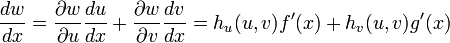$\frac{dw}{dx} = \frac{\partial w}{\partial u}\frac{du}{dx} + \frac{\partial w}{\partial v} \frac{dv}{dx} = h_u(u,v)f'(x) + h_v(u,v)g'(x)$
Pure functional notation (generic point) Suppose$f,g$ are both functions of one variable and$h$ is a function of two variables. Consider the function:$H(x) := h(f(x),g(x))$
Then, we have$H'(x) = h_1(f(x),g(x))f'(x) + h_2(f(x),g(x))g'(x)$ where$h_1, h_2$ denote respectively the partial derivatives with respect to the first and second coordinates.
Pure dependent variable notation (generic point) Suppose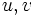$u,v$ are variables functionally dependent on$x$ and$w$ is a variable functionally dependent on both$u$ and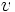$v$. Then,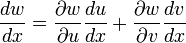$\frac{dw}{dx} = \frac{\partial w}{\partial u}\frac{du}{dx} + \frac{\partial w}{\partial v} \frac{dv}{dx}$

Conceptual statement for a two-step composition

Consider a situation where we have three kinds of variables:

• Independent input variables
• Dependent intermediate variables, each of which is a function of the input variables.
• Dependent output variables, each of which is a function of the intermediate variables.

Then, we have:

Partial derivative of any dependent output variable with respect to any independent input variable =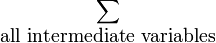$\sum_{\mbox{all intermediate variables}}$ Partial derivative of output variable with respect to input variable via intermediate variable =$\sum_{\mbox{all intermediate variables}}$ (Partial derivative of output variable with respect to intermediate variable) times (Partial derivative of intermediate variable with respect to input variable)

In other words, we get in general a sum of products, each product being of two partial derivatives involving the intermediate variable.

As noted above, in those cases where the functions involved have only one input, the partial derivative becomes an ordinary derivative.

Statement with symbols for a two-step composition

Suppose we have:

• Independent input variables$x_1,x_2,\dots,x_n$
• Dependent intermediate variables,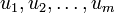$u_1,u_2,\dots,u_m$, each of which is a function of$x_1,x_2,\dots,x_n$.
• Dependent output variables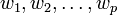$w_1,w_2,\dots,w_p$, each of which of a function of$u_1,u_2,\dots,u_m$.

Then, for any$i \in \{ 1,2,3,\dots,p \}$ and$j \in \{ 1,2,3,\dots,n\}$, we have: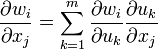$\frac{\partial w_i}{\partial x_j} = \sum_{k=1}^m \frac{\partial w_i}{\partial u_k} \frac{\partial u_k}{\partial x_j}$